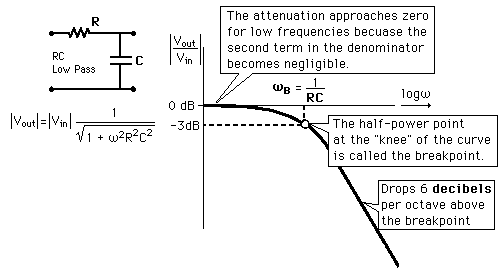# 3dB frequency / knee frquency

Status
Not open for further replies.

#### chuan556

##### Member level 2may i ask how we relate our square wave to the 3dB frequency?
and where can i find the detail or explaination for this?

#### v_c

##### Advanced Member level 2I don't understand what you are trying ask? Can you be more clear.

3dB point refers to a frequency response plot of a transfer function where the gain falls 3dB below the maximum level.

A square wave is in the time domain -- what does this have to do with 3db?
A square wave will have odd harmonics, etc. Are you asking for the Fourier Series coefficients for the square wave.

Best regards,
v_c

#### chuan556

##### Member level 2may you explain more detail on 3dB point?or can i have any example or reference?
sorry i am beginner,thanks

#### jallem

##### Advanced Member level 1see section 1.1 pg 3 of High-Speed Digital Design
by Johnson/Graham

#### v_c

##### Advanced Member level 2To really understand the 3dB frequency (sometimes called corner frequency or break frequency), you have to understand transfer functions and maybe Bode plots.
I suggest you take a look at this page https://www.swarthmore.edu/NatSci/echeeve1/Ref/E72WhaKnow/WhaKnowSys.html
If you look at the section under First Order Systems you will see the definition. Also look under Second Order Systems for more information.

Best regards,
v_c

#### gigle

##### Junior Member level 1You can construc the square wave from sine wave. In this case you need infinite number of frequency components of sine wave, therefore the bandwidth of ideal square wave is infinity.

Many digital communication books are dealing with the concept of data rate and band width.

And also you can find the "Fourier series" in many mathmatic books or communication books.

#### v_c

##### Advanced Member level 2gigle said:
You can construc the square wave from sine wave. In this case you need infinite number of frequency components of sine wave, therefore the bandwidth of ideal square wave is infinity.

Many digital communication books are dealing with the concept of data rate and band width.

And also you can find the "Fourier series" in many mathmatic books or communication books.

gigle,

you are right about infinite number of sinewaves needed to reconstruct the square wave. In fact for a square wave of amplitude $\pm V_s$ at some frequency $f_s$, you need infinite number of odd harmonics each with amplitude $\frac{4 V_s}{n \pi}$ at each frequency $n f_s$ where $n = 1, 3, 5, \ldots$. So if we consider the amplitude at $n=1$ as our reference, the third harmonic (which is the next highest non-zero harmonic) will be 1/3 of this value. And a factor of 1/3 translates into $20 \log (1/3) = -9.54dB$, so the 3dB point (actualy -3dB point) will be somewhere between the fundamental and the third harmonic. But note that there will be no component of the square wave there.

The interpretation for 3dB point for a waveform is slightly different. Given a 3dB point of a waveform means that 1/2 the power of the waveform is contained below the 3dB point the other is contained above.Best regards,
v_c

Added after 14 minutes:

I think I am describing the 3dB point above. Apparently, knee frequency means something different -- I did not know that. When I looked into the
Johnson & Graham's book (as jallem suggested), I did see a different definition that has do with the spectrum of clock signals with finite rise/fall times.

The book is on EDAboard -- take a look here

Best regards,
v_c

#### jallem

##### Advanced Member level 1The knee frequency is an indication of how fast the signal
change( a square wave, I mean) from 0 to 1. The ratio knee/3-dB frequencies
is an indication how much time is left of margin. In other words,
if you have a digital signal which as 400 ps period but you have a rise time of
100 ps then you have 300 ps of margin. If your setup or hold time
is greater than that then you need to decrease the clock rate. If you add
some others restrictions as phase noise/jitter for example then you
margin is further reduced. In high speed digital design you want a clock as fast
as you can but there is a limit, the signal must be stable in other to be error free.

Added after 13 minutes:

I forgot.... even if you have a driver and a receiver that they have
enough margin to talk to each other, they need to be connected by
a physical medium, the channel(coax cable, RS232, fiber,etc). If this
channel has restriction regarding bandwidth , in other words, the
3-dB bandwidth of the channel is lower than the knee frequency of the
signal, then the rise time of the signal will be increased and lowering your
margin. If you add ringing(mismatching) and settling time to it you will
have further reduction in margin.

#### chuan556

##### Member level 2if we pump in a square signal how we get the knee frequency?

#### jallem

##### Advanced Member level 1What do you mean? How to calculate it?

#### chuan556

##### Member level 2ya i mean how to calculate it,got any formula or.....

#### jallem

##### Advanced Member level 1ya i mean how to calculate it,got any formula or.....
see section 1.1 pg 3 of High-Speed Digital Design
by Johnson/Graham

Status
Not open for further replies.